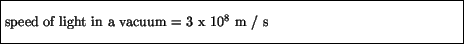Next: Electromagnetic spectrum Up: Light Previous: The Doppler effect

# Electromagnetic waves

Most waves we are familiar with involve some sort of medium which supports the waving'' - sound waves propagate via oscillations of molecules (air, water, etc.), water waves exist on water, and so on. The natural question arises: what medium is needed for light waves? For years scientists looked for such a medium, which was called ether, without success. It is now believed that light can propagate in a vacuum, and so does not need a medium to exist.

Strong support for this view came from an unexpected source - electricity and magnetism. We saw in the last chapter that there are some strong connections between electricity and magnetism: electric currents give rise to magnetic fields, and changing magnetic fields induce currents. These effects are summarized by what are known as Maxwell's equations, which are a set of mathematical relations which, given any source of charges and/or currents, allow one to solve in principle for the resulting electric and magnetic fields. These equations are a great triumph in science, and are extremely well verified experimentally. Now, if one examines these equations in a vacuum (i. e., in the absence of any source of charge and current), one finds a non-trivial solution emerges. This solution mathematically is a simple wave, just like the type we have been considering, and has a speed given completely in terms of the parameters arising in Maxwell's equations. This speed turns out to be numerically equal to the speed of light in a vacuum:What this means is that light is interpreted as a set of oscillating electric and magnetic fields, which can and do exist in a vacuum.Next: Electromagnetic spectrum Up: Light Previous: The Doppler effect
modtech@theory.uwinnipeg.ca
1999-09-29